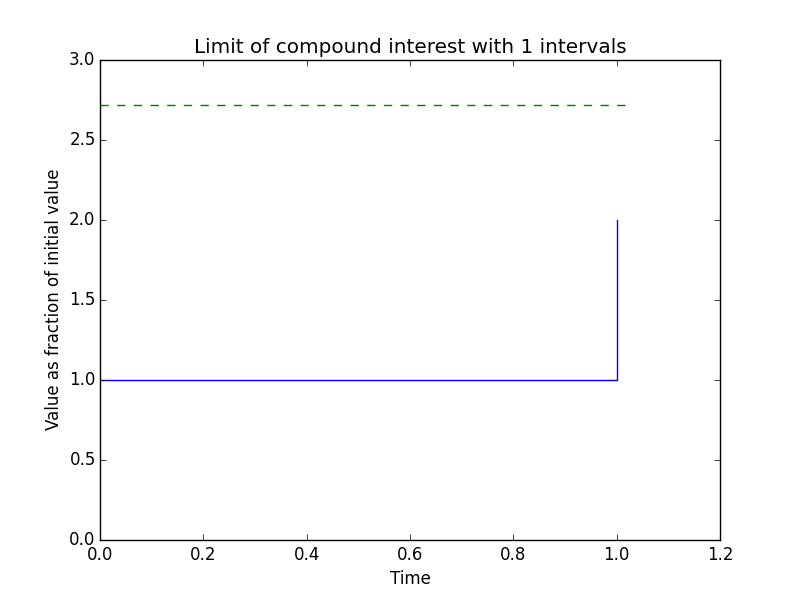I saw this post on /r/math today, which asks if there is an intuitive illustration of the value of the constant e=2.7183… There are a few ways of showing this, including the definition of e as the upper limit of $$\int_{1}^{e}{\frac{1}{x} dx}=1$$. This is definitely true, but isn’t particularly satisfying - you can see e on the graph, sure, but where does that value come from? There’s also the infinite series $$e=\sum_{n=1}^{\infty}{\frac{1}{n!}}$$, but again it’s not too intuitive.

I remember watching this particularly catchy video on the constant e some time ago, and the compound interest graph 18 seconds into the video caught my eye - you can see how the value tends to e as the number of intervals increases. Not wanting to rip the visualisation straight from the video I decided to create a visualisation myself. That’s probably what you came here to see, so here it is:## the code

I used python and matplotlib to create this visualisation. I’ve never used matplotlib before, and I scarcely use Python either, so this is somewhat cobbled-together. Here it is:

There isn’t a great deal to describe - I’ve detailed the repetition of animation frames in the comments of the code. You’ll need matplotlib (of course) to plot the visualisation and you’ll also need to have ImageMagick to create the animated GIF. Alternatively, you could use FFmpeg and change the final statement to generate an MP4 instead.

The code is available on Gist, too.